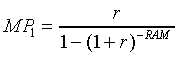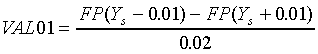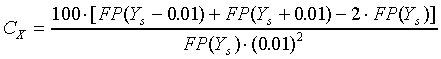DURATION

Modified duration, DMod, for a single-tranche MBS maturing at M can be calculated using the formula:CONVEXITY

Similarly, convexity, Cx, can be calculated using the formula:VAL01

VAL01, the dollar value of a 100 basis point change in yield, can be calculated as follows:where

FP(Ys)=price plus accrued calculated at a semi-annual yield of Ys;

Duration and convexity can also be calculated using shocks to the yield curve:;Note that, in the numerators of equations 20 through 22, accrued interest cancels out; thus, market prices could be used. However, in the denominators of 21 and 22, the full price including accrued interest must be used.

Date Published: March 31, 2018